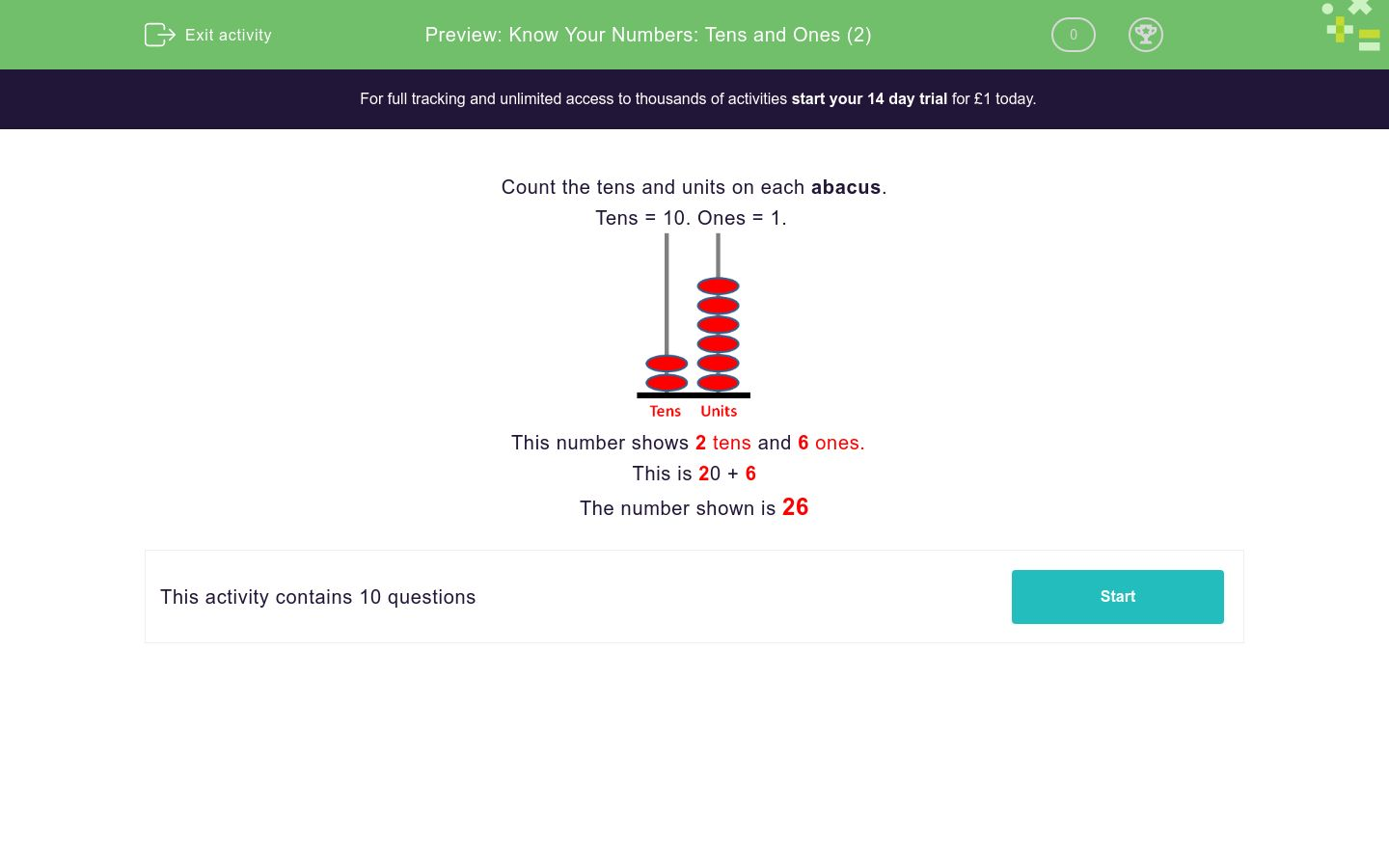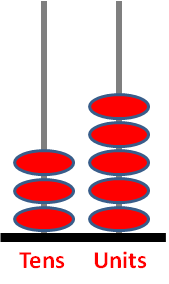# Know Your Numbers: Tens and Ones (2)

In this worksheet, students write down the number shown on the tens and ones abacus.Key stage:  KS 1

Curriculum topic:   Number: Number and Place Value

Curriculum subtopic:   Recognise Tens and Ones

Difficulty level:### QUESTION 1 of 10

Count the tens and units on each abacus.

Tens = 10. Ones = 1.This number shows 2 tens and 6 ones.

This is 20 + 6

The number shown is 26

Count the tens and ones. Type in the total.Count the tens and ones. Type in the total.Count the tens and ones. Type in the total.Count the tens and ones. Type in the total.Count the tens and ones. Type in the total.Count the tens and ones. Type in the total.Count the tens and ones. Type in the total.Count the tens and ones. Type in the total.Count the tens and ones. Type in the total.Count the tens and ones. Type in the total.• Question 1

Count the tens and ones. Type in the total.23
EDDIE SAYS
2 tens = 20
20 + 3 ones = 23
• Question 2

Count the tens and ones. Type in the total.43
EDDIE SAYS
4 tens = 40
40 + 3 = 43
• Question 3

Count the tens and ones. Type in the total.75
EDDIE SAYS
7 tens = 70
70 + 5 = 75
• Question 4

Count the tens and ones. Type in the total.21
EDDIE SAYS
2 tens = 20
20 + 1 = 21
• Question 5

Count the tens and ones. Type in the total.20
EDDIE SAYS
2 tens = 20
So the total is 20.
There are no ones.
• Question 6

Count the tens and ones. Type in the total.4
EDDIE SAYS
There are no tens.
But there are 4 ones.
• Question 7

Count the tens and ones. Type in the total.35
EDDIE SAYS
3 tens = 30
30 + 5 ones = 35
• Question 8

Count the tens and ones. Type in the total.26
EDDIE SAYS
2 tens = 20
20 + 6 ones = 26
• Question 9

Count the tens and ones. Type in the total.83
EDDIE SAYS
8 tens = 80
80 + 3 ones = 83
• Question 10

Count the tens and ones. Type in the total.38
EDDIE SAYS
3 tens = 30
30 + 8 = 38
---- OR ----

Sign up for a £1 trial so you can track and measure your child's progress on this activity.

### What is EdPlace?

We're your National Curriculum aligned online education content provider helping each child succeed in English, maths and science from year 1 to GCSE. With an EdPlace account you’ll be able to track and measure progress, helping each child achieve their best. We build confidence and attainment by personalising each child’s learning at a level that suits them.

Get started